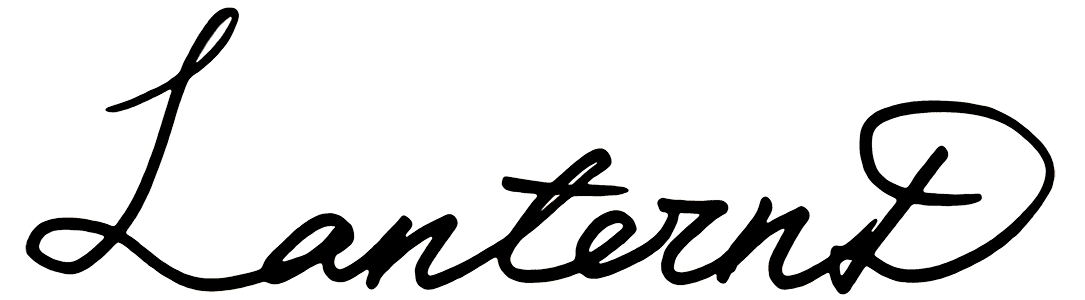# 123456789与100

在StackExchange看到一个有意思的问题：在1 2 3 4 5 6 7 8 9 = 100之间加上运算符号，使最后的表达式成立，但是让算符尽可能少。这里摘录一些有意思的结果。

## 题目

原问题：

The sequence of numbers 1 2 3 4 5 6 7 8 9 has the property that you can insert mathematical operators in between the numbers from 1 to 9 and make the expression evaluate to 100. For example:

$1+2+3+4+5+6+7+8×9=100$

There are possibly hundreds of solutions to this problem, involving different varieties of operators. What is the expression with the fewest number of operators inserted (out of the set +,−,×,÷ and maybe √ and !) that evaluates to 100?

在序列1 2 3 4 5 6 7 8 9之间插入数学运算符，使得整个式子的结果等于100。如果考虑到不同的运算符组合，该问题可能有数百种答案，哪一个表达式所需的操作符最少？（可以使用$+-\times\div$甚至$\sqrt{}$和$!$）

## 一些答案

以下是一些网友们的回答：

### 先晒奇葩

• $123456789！=100$

仅一个算符！懂编程的朋友都知道，在大部分语言中「!=」是「不等于」的意思，放在条件语句中进行判断操作。这里「猥琐」地加了个叹号，让表达式「成立」了。我可能会承认这是个答案，但肯定不是提问者想要的答案，于是继续往下看。

• $123456789=/=100$

不得不说这个更猥琐。加上「=/」以后，和后面的等号合成了「$\neq$」。好吧，这肯定也是不符合要求的，不多说了。

### 7个算符

• $- ( 12 + 34 - 56 ) \times ( -7 + 8 + 9 ) = 100$

7个算符不包括括号，看起来颇有点意思。$-10\times(-10)=100$，毫无疑问这是可行的。这是来自某网友绞尽脑汁想出来的回答。

### 4个算符

• $123 + 45 - 67 + 8 - 9 = 100$

4个算符的回答貌似属于跨越式的发展。形式比之前7个算符更加简洁。至于是怎么得到的，下文见分晓。

• $123 + 4- 5 + 67 - 89 = 100$

和上面的式子一样来自同一个用户。

### 3个算符

• $(1234\mod567)\mod8^9=100$

这里巧妙的引进了同余运算，简单一些来说就是求余数。该运算在编程中为百分号「$\%$」。所以上面的式子也可以写为：$(1234\%567)\%8^9=100$。还有一个算符自然就是乘方运算「$^$」，是否一目了然了呢。

其实令我惊讶的是$1234\div567$的余数竟然刚好是$100$。不知道是有规律可循还是碰巧。

• $123-45-67+89 = 100$

这是只用$+-\times\div$能得到的最少算符表达式了，甚至都没用$\times\div$。不得不说是个非常不错的结果。这也是得票第二多的结果。

### 2个算符(奇葩？)

• $1^{23456789}=10^0$

放到最后的是最高票的答案。只用两个乘方算符$^$。左右都等于$1$。当然这个也有争议，有评论指出这个式子给右边的$100$做了手脚，得到的并不是理想的答案，性质和前面的「$!=$」一样。

谁知道呢，可能不是提问者想要的，但是也是可以让许多人觉得合理的。

## Geek

上面卖个关子没有说明只用4个算符的答案是怎么来的。现在贴在这里。那位用户Engineer Toast自己编了个程序，用穷举法得到了所有的可能性。不过他只用了$+-\times\div$、$^$和小数点。

直接把代码贴上来了。据称是在Excel中使用的VBA。我挺欣赏这种Geek的行为，为了解决一个问题，提供「完全」、「终极」的一大类解决方案（虽然大概是暴力穷举法）。代码我也是大概看看，更详细的解释我也就不提供了。

Sub doTheMath()

Const op As String = " +-*/.^"
Dim s As String
Dim a, b, c, d, e, f, g, h
Dim i, j
Dim v

'Loop all possibilities
i = 2
j = 1
For a = 1 To Len(op):    For b = 1 To Len(op):    For c = 1 To Len(op):    For d = 1 To Len(op)
For e = 1 To Len(op):    For f = 1 To Len(op):    For g = 1 To Len(op):    For h = 1 To Len(op)
s = 1 & Mid(op, a, 1) & 2 & Mid(op, b, 1) & 3 & Mid(op, c, 1) & 4 & Mid(op, d, 1) & 5 & _
Mid(op, e, 1) & 6 & Mid(op, f, 1) & 7 & Mid(op, g, 1) & 8 & Mid(op, h, 1) & 9
v = Application.Evaluate(Replace(s, " ", ""))
If CStr(v) = CStr(100) Then
Cells(i, 1).Value = s
Cells(i, 2).Value = v
i = i + 1
End If
j = j + 1
If j > 1000000 Then
ThisWorkbook.Save
j = 1
End If
Next:    Next:    Next:    Next:    Next:    Next:    Next:    Next

End Sub


这个是他得到的结果：

Operators Solutions
0 0
1 0
2 0
3 1
4 2
5 9
6 57
7 104
8 42

对于3个算符只有一种可能，上文已经写过了。4个算符有2种方案，之后还有更多的算符，但是可以想象，算符越多不意味着会有更多的结果。7个算符的方案是最多的，多达104。

## 后记

乍一看这个问题和我们平常玩的24点有点像，从扑克牌中抽4张牌，通过各种运算使最后的答案等于24。这个游戏对开发智力还是蛮有好处的，可以提高算术思维的能力。但是今天的问题和24点也有所不同：

• 必须按顺序 　提问者已把顺序给定，而在24点中抽到的的数字的顺序是可以随意调换的。
• 可以把数字组合起来 　题目中的数字可以随便组合到一起，将固定组合最大化利用。

• 需要算符最少 　24点中毫无疑问至少需要三个算符，有可能还要更多，只要能够算出24可以「不择手段」。

总之，无意中看到的这个问题觉得蛮有意思的。所以拿出来分享分享。要说我自己还真没有想出合适的答案，大概已经被上面的内容包括了吧。

不知各位有没有自己的答案呢。

LanternD